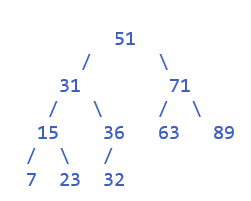## Binary Tree sorting in java

Category : Data-structures-algorithms | Sub Category : Sorting Algorithms | By Runner Dev Last updated: 2020-10-31 19:11:42 Viewed : 197

Binary Tree sorting

A binary tree is a data structure in which an element has two successors(children). The left child is usually smaller than the parent, and the right child is usually bigger.

Worst complexity: n*log(n) (balanced)

Average complexity: n*log(n)

Best complexity: n*log(n)

Space complexity: n

Input:

51, 31, 71, 15, 7, 63, 23, 36, 89, 23, 32Here , 51 root node

Step 1:    31 < 51 (less than the current node move to the left)

Step 2:    71 >51  (greater than the current node move to the right)

Step 3:   15 <51 and 15<31  (less than the current node move to the left to the left)

Step 4:    7 < 51 and 7<31 and 7<15 (less than the current node move to the left to the left to the left)

Step 5:    63>51 and 63<51 (less than the current node move to the right to the left)

Step 6:   23<51 and 23 < 31  and 15> 23 (less than the current node move to the left to the left to the right)

..vice versa elements will be added into the Binary tree

TreeSort.java

` package runnerdev; `

import java.util.Arrays;

public class TreeSort {

/** A node class representing each node in the binary search tree. */

static class Node {

int value;

Node left;

Node right;

public Node(int value) {

this.value = value;

left = null;

right = null;

}

}

Node node;

TreeSort() {

node = null;

}

/* insert a new key in Binary Sort Tree */

Node insert(Node node, int value) {

if (node == null) {

node = new Node(value);

return node;

}

/** If new node’s value is less than the current node move to the left. **/

if (value < node.value)

node.left = insert(node.left, value);

/** If new node’s value is greater than the current node move to the right. **/

else if (value > node.value)

node.right = insert(node.right, value);

return node;

}

// For traversing in order

public void inOrderAsc(Node node) {

if (node != null) {

inOrderAsc(node.left);

System.out.print(node.value + " ");

inOrderAsc(node.right);

}

}

public void inOrderDesc(Node node) {

if (node != null) {

inOrderDesc(node.right);

System.out.print(node.value + " ");

inOrderDesc(node.left);

}

}

/** Insert Array **/

void treeInsert(int array[]) {

for (int number : array) {

node = insert(node, number);

}

}

/** Binary tree create **/

public static TreeSort create() {

TreeSort tree = new TreeSort();

Node node = new Node(51);

tree.node = node;

tree.node.left = new Node(31);

tree.node.left.left = new Node(15);

tree.node.left.left.left = new Node(7);

tree.node.left.right = new Node(36);

tree.node.left.right.left = new Node(32);

tree.node.right = new Node(71);

tree.node.right.left = new Node(63);

return tree;

}

/**

* traverse the binary tree on InOrder traversal algorithm

*/

public void inOrder() {

inOrderAsc(node);

}

public static void main(String[] args) {

/** create Binary Tree **/

TreeSort treeSort = TreeSort.create();

// traversing binary tree using recursion

System.out.println("printing nodes of binary tree in Ascending order :");

treeSort.inOrder();

TreeSort tree = new TreeSort();

int array[] = {51, 31, 71, 15, 7, 63, 23, 36, 89, 23, 32 };

System.out.println(" Original array: " + Arrays.toString(array));

tree.treeInsert(array);

System.out.println("Ascending Order : ");

tree.inOrderAsc(tree.node);

System.out.println(" Descending Order : ");

tree.inOrderDesc(tree.node);

}

}

OutPut:

printing nodes of binary tree in Ascending order : 7 15 31 32 36 51 63 71

Original array: [51, 31, 71, 15, 7, 63, 23, 36, 89, 23, 32]

Ascending Order : 7 15 23 31 32 36 51 63 71 89

Descending Order : 89 71 63 51 36 32 31 23 15 7